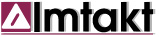Solution Dilution Calculator
This calculator is a tool for an accurate solution dilution.
Input an initial concentration value/unit, initial or final volume, select unit you want to convert to, then press “CALCULATE”.
Solution density is assumed to be “1”.
Enter the molar mass for conversions between molar concentration and %.

Molar Mass / Mol Wt:  g/mol

Initial Concn:    UNIT

Final Concn:   UNIT

Initial VOL:    µL mL L
Final VOL:    µL mL L

VOL Difference:

 Copyright: I.YAZAWA / Imtakt Corp.